# Image Processing Tutorial Using scikit-image - Thresholding

Betul Mescioglu

## Thresholding:

Thresholding is a type of image segmentation where we change the value of pixels based on a criteria (threshold) for analysis purposes. A threshold in image processing could be likened to height sticks used in amusement parks. Any child shorter than the stick is denied, whereas the rest is allowed to enjoy the ride. Likewise, each pixel's value is compared to a pre-determined threshold, and based on that each pixel value is changed. We use this method to select objects of interest, while ignoring the rest.

``````#Import necessary libraries
import skimage.io
import matplotlib.pyplot as plt
from skimage.filters import gaussian
import numpy as np
skimage.io.imshow(musical)
plt.title('Original Image')
plt.axis('off')``````
`(-0.5, 1299.5, 1299.5, -0.5)`Here, we are interested in the musical instruments only. So, we will turn on the pixels belonging to the musical instruments, while turning off the rest of the image pixels.

First, we will convert the image to grayscale, and apply a Gaussian filter and thresholding. The pixels less than the threshold value would be turned off. Since we do not want to omit any valuable data, we need to come up with a good threshold value. For that, we would have to look at the histogram of the grayscale image.

``````#Convert image to grayscale
gray_musical = skimage.color.rgb2gray(musical)
#Denoise the image
blurred_musical = skimage.filters.gaussian(gray_musical, sigma=1.0)
#Plot the result
plt.imshow(blurred_musical, cmap='gray')
plt.axis('off')
plt.title('Denoised Grayscale Image')
plt.show()``````Let's plot the histogram of the grayscale image by using numpy's histogram() function:

``````#Create and plot the histogram:
histogram, bin_edges = np.histogram(blurred_musical, bins=256, range=(0.0, 1.0))
plt.plot(bin_edges[0:-1], histogram)
plt.title('Grayscale Histogram')
plt.xlabel('Grayscale value')
plt.ylabel('Number of Pixels')
plt.xlim([0, 1.0])
plt.show()``````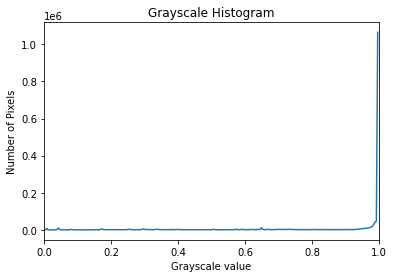Since the background of the image is white, we see a peak at 1. We see some waves before the peak, but they are barely visible. Let's ignore the peak so we could zoom in on those peaks:

``````#Draw the histogram without the peak at 1
plt.plot(bin_edges[0:-1], histogram)
plt.title('Grayscale Histogram')
plt.xlabel('Grayscale value')
plt.ylabel('Number of Pixels')
plt.xlim([0, 0.9])
plt.ylim([0,20000])
plt.show()``````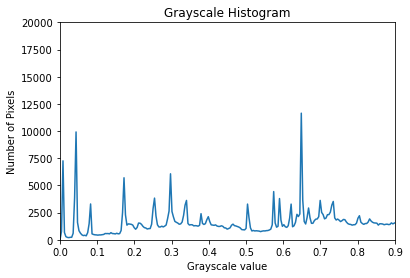Now, we see lots of important data that we do not want to miss out on. But after 0.85, the graph flat-lines a bit before peaking at 1. So, it seems like if we choose our threshold around the value of 0.85, we will not miss any musical instruments' pixels but will turn off the white background. Let's create a binary mask that will black out anything with a value greater than 0.85.

``````#Create a binary mask with threshold value of 0.85
threshold = 0.85
plt.axis('off')
plt.show()``````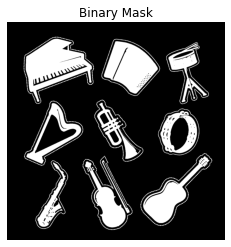We created a black and white (binary) mask for the image. Let's apply that mask to the image to bring only the musical instruments to the foreground and black out the rest of the image.

``````#Create a mask with all zero values
selection = np.zeros_like(musical)
#Change mask's pixel values to show through the colors of the instruments
plt.imshow(selection)
plt.axis('off')
plt.show()``````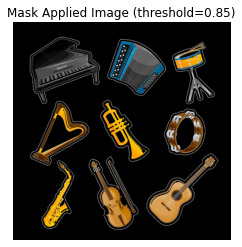So far, we practiced detecting an appropriate threshold value by inspecting the histogram.

We can also let skimage find the best threshold automatically.

In the following section, we will find the threshold by using Otsu's method. Then, we will use try_all_threshold() function which goes through many different thresholding algorithms to produce thresholds. Based on these results we can decide which algorithm works best for the task at hand.

The Otsu's Method:

``````#Find Otsu threshold:
threshold_otsu = skimage.filters.threshold_otsu(blurred_musical)
print('Threshold found with Otsu\'s method is : {}'.format(round(threshold_otsu, 3)))``````
```Threshold found with Otsu's method is : 0.667
```

Now we will create a binary mask by using this threshold value:

``````#Creating a binary mask:
plt.title('Binary Mask Created with Otsu\'s Method\n (threshold=0.667)')
plt.axis('off')
plt.show()``````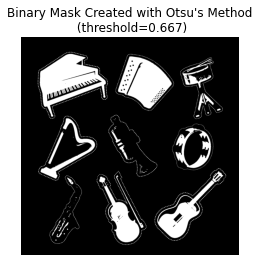Let's apply that binary mask to the original image to bring the musical instruments to the foreground.

``````#Create a mask with all zero values
selection_otsu = np.zeros_like(musical)
#Change mask's pixel values to show through the colors of the instruments
plt.imshow(selection_otsu)
plt.axis('off')
plt.show()``````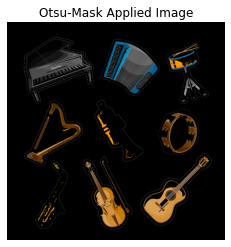Let's compare the result of Otsu's method with the one we obtained by selecting a threshold:

``````fig, ax = plt.subplots(ncols=2, sharex=True, figsize=(10,8))
ax.imshow(selection)
ax.set_title('Threshold Selected by User (0.85)')
ax.imshow(selection_otsu)
ax.set_title('Otsu\'s Threshold (0.667)')
for a in ax:
a.axis('off')``````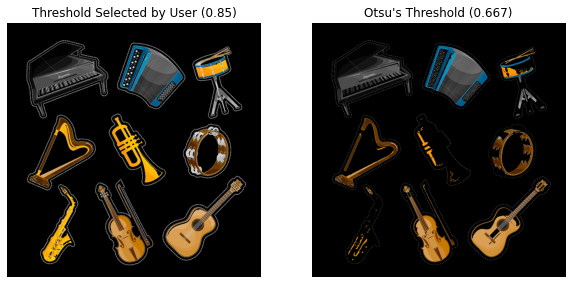With Otsu's method we were able to get rid of the outline around the musical instruments at the expense of losing valuable details of the musical instruments. Let's say our goal is to count the number of the musical instruments, then Otsu's method provides enough data at a lot less computational expense. However, if our goal involves having as much details of the objects as possible, then user defined threshold is the option to go with.

"Try All" method:

Let's use try_all_threshold() function from skimage.filters to detect threshold values calculated by seven different algorithms.

``````#import necessary function:
from skimage.filters import try_all_threshold
fig, ax = try_all_threshold(blurred_musical, figsize=(14, 12), verbose=False)
plt.tight_layout()
plt.show()``````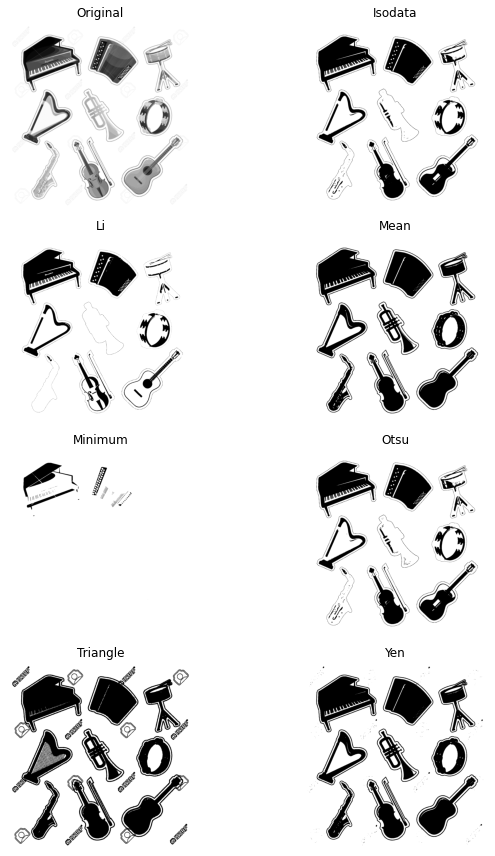Let's write a function that will go through those thresholding algorithms, create a mask with the newly created threshold and apply it to the original image.

``````#Import necessary thresholding functions:
from skimage.filters import (threshold_li, threshold_minimum,
threshold_triangle, threshold_isodata,
threshold_mean, threshold_otsu, threshold_yen)
def apply_thresh_algorithm(image):
'''
This function takes in an image, turns it to grayscale,
denoises it with a Gaussian filter.
It then calculates seven threshold values
corresponding to seven different algorithms for this
grayscale image, creates masks using those values
and finally applies those masks to the original image.
All masked images are plotted along with the original image
for comparison purposes.
'''
grayscale_image = skimage.color.rgb2gray(image)
blurred_image = skimage.filters.gaussian(grayscale_image)
thresh = [threshold_li, threshold_minimum, threshold_triangle,
threshold_isodata, threshold_mean, threshold_otsu, threshold_yen]
algorithm_name = []
#Plot the Original and masked images:
fig, ax = plt.subplots(nrows=2, ncols=4, figsize=(18, 10), sharex=True, sharey=True)
ax =ax.ravel()
ax.imshow(image)
ax.set_title('Original Image')
count = 0
for i in thresh:
count += 1
algorithm_name.append((str(i).split('_')).split(' ').title())
thresh = i(blurred_image)